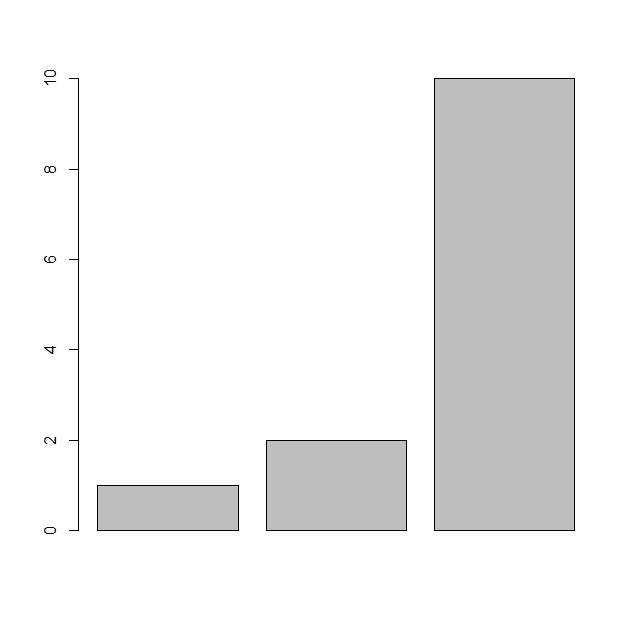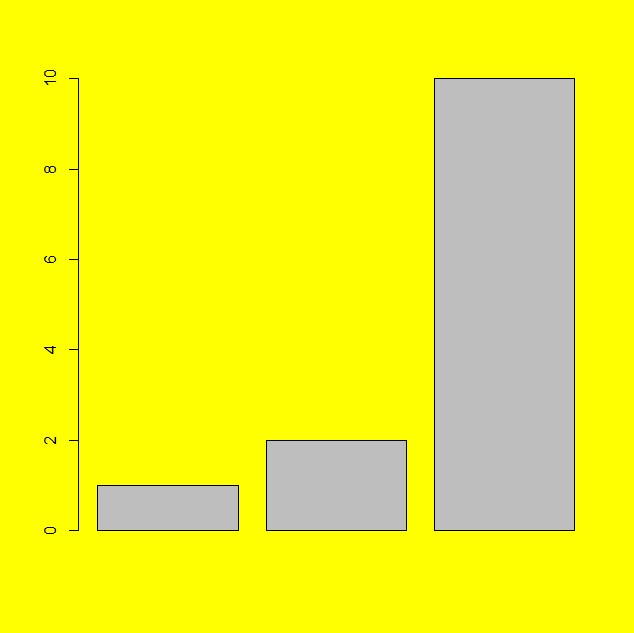# How to change the background color of base R plot region?

To change the background color of base R plot region, we can follow the below steps −

• First of all, create a plot in base R to understand the difference.

• Then, use par function with bg argument where you can put color name and then create the same plot.

## Example

#### Create the plot

Let’s create a barplot in base R as shown below −

x<-sample(1:10,3)
barplot(x)

## OutputCreate the plot with changed background color

Using par function with bg argument having yellow color and then creating the barplot −

x<-sample(1:10,3)
par(bg="yellow")
barplot(x)

## Output